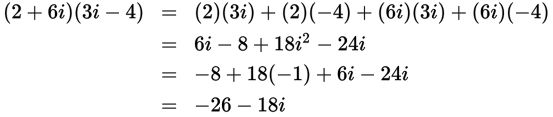# SAT Math Multiple Choice Question 944: Answer and Explanation

### Test Information

Question: 944

12. Which of the following shows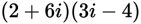written as a complex number in the form a + bi? (Note: i2 = -1)

• A.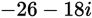• B.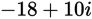• C.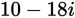• D.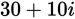Explanation:

A

Difficulty: Easy

Category: Additional Topics in Math / Imaginary Numbers

Strategic Advice: You can treat complex numbers as binomials and use FOIL to multiply them. Also remember that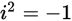.

Getting to the Answer: Use FOIL to multiply the two complex numbers, and then combine like terms (the real parts and the imaginary parts):